# Python Data Essentials - Matplotlib and Seaborn

## A beginners guide.

Posted by Craig Johnston on Sunday, July 8, 2018
##### SHARE

There is an overwhelming number of options for developers needing to provide data visualization. The most popular library for data visualization in Python is Matplotlib, and built directly on top of Matplotlib is Seaborn. The Seaborn library is “tightly integrated with the PyData stack, including support for numpy and pandas data structures and statistical routines from scipy and statsmodels.”

This article is only intended to get you started with Matplotlib and Seaborn. Both libraries have extensive and mature documentation.

## Data Visualization Landscape

For large scale business analytics and analysis, there are commercial tools such as Tableau or Pentaho, they look great and have ample features, yet they have high costs and implementation commitments, not to mention vendor lock-in. Commercial applications and large visualization platforms focus your development around their feature set.

For front-end interactive developers there are open source javascript libraries like d3js/c3js. However, these solutions are often overkill for simple, static bar charts, histograms and plots; they are also problematic for print and PDF.

It’s common in many of my projects to generate static reports. These static reports are designed in HTML and use a tool I build called txpdf, a Webpage to PDF Microservice use to convert them to PDF and email them to the appropriate stakeholder. I use serverless functions with numpy and pandas to processes many forms of data, which makes Matplotlib with Seaborn an excellent tool for adding data visualization to these reports.

## Notes on Data

In the following examples, I’ll be making up some simple data with small datasets for brevity in the demonstration. However, if you are looking to experiment with a large variety of publicly available datasets, I recommend you visit kaggle.

## Python Libraries

This article is written using Juypter Notebooks installed and running under Anaconda. I recommend this setup for experimenting and learning. In the examples below I use Python 3 with the libraries numpy, pandas, Matplotlib and Seaborn. The Anaconda command `conda list` shows me the available libraries and their installed versions. The line `%matplotlib inline` instructs Juypter Notebooks to display Matplotlib output inline, rather than rendered to a file. If you are running Anaconda you likely have all the required libraries.

``````import numpy as np
import pandas as pd
import matplotlib.pyplot as plt
import seaborn as sb
import warnings
warnings.filterwarnings('ignore')

%matplotlib inline

!conda list numpy
!conda list pandas
!conda list matplotlib
!conda list seaborn

``````
``````# packages in environment at /Users/cjimti/anaconda3:
#
# Name                    Version                   Build  Channel
numpy                     1.13.3           py36ha9ae307_4
numpy-base                1.14.3           py36ha9ae307_2
numpydoc                  0.8.0                    py36_0
# packages in environment at /Users/cjimti/anaconda3:
#
# Name                    Version                   Build  Channel
pandas                    0.22.0           py36h0a44026_0
# packages in environment at /Users/cjimti/anaconda3:
#
# Name                    Version                   Build  Channel
matplotlib                2.2.2            py36ha7267d0_0
# packages in environment at /Users/cjimti/anaconda3:
#
# Name                    Version                   Build  Channel
seaborn                   0.8.1            py36h595ecd9_0
``````

## Dataset

Below I set up a simple data set in a Pandas using a DataFrame.

``````ai = {'wintermute': pd.Series(data = [1, 4030, 100, 100], index = ['type','id','dangerous','intellect']),
'hal': pd.Series(data = [1, 70, 100, 90, 20], index = ['type','id','intellect','dangerous','bending']),
'rachael': pd.Series(data = [2, 7192, 80, 40], index = ['type','id','intellect','dangerous']),
'johnny 5': pd.Series(data = [2, 836, 12, 5, 2], index = ['type','id','dangerous','humor','bending']),
'ava': pd.Series(data = [2, 9272, 80, 95, 10], index = ['type','id','intellect','dangerous','bending']),
'bender': pd.Series(data = [2, 7912, 20, 50, 90, 100], index = ['type','id','intellect','dangerous','humor','bending'])}

df_ai = pd.DataFrame(ai)

# replace NaNs with ones assuming our bots have at least
# some capability in each category
df_ai.fillna(1, inplace=True)

# transpose
df_ai_t = df_ai.T

# convert to intergers
df_ai_t = df_ai_t.astype(int)

# let juypter output a nice html table
df_ai_t
``````
bending dangerous humor id intellect type
ava 10 95 1 9272 80 2
bender 100 50 90 7912 20 2
hal 20 90 1 70 100 1
johnny 5 2 12 5 836 1 2
rachael 1 40 1 7192 80 2
wintermute 1 100 1 4030 100 1

## Bar Charts

Seaborn’s seaborn.countplot delivers nice and simple quantitative representations of qualitative data sets.

### seaborn.countplot

We have two types of AI bots, three of type 1 and 2 of type 2 using seaborn.countplot we can see a quantitative comparison.

``````sb.countplot(data = df_ai_t, x = 'type'); # the semi-colon supresses object output info
``````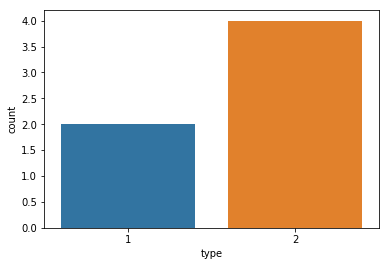Automatic coloring of the data can lead to the unintended highlighting of data. If we want we only want to present the value differences, it is better to have a uniform color.

We can get a list of colors from the Seaborn color pallet and set the color with one of the tuples.

``````sb.color_palette()
``````
``````[(0.12156862745098039, 0.4666666666666667, 0.7058823529411765),
(1.0, 0.4980392156862745, 0.054901960784313725),
(0.17254901960784313, 0.6274509803921569, 0.17254901960784313),
(0.8392156862745098, 0.15294117647058825, 0.1568627450980392),
(0.5803921568627451, 0.403921568627451, 0.7411764705882353),
(0.5490196078431373, 0.33725490196078434, 0.29411764705882354),
(0.8901960784313725, 0.4666666666666667, 0.7607843137254902),
(0.4980392156862745, 0.4980392156862745, 0.4980392156862745),
(0.7372549019607844, 0.7411764705882353, 0.13333333333333333),
(0.09019607843137255, 0.7450980392156863, 0.8117647058823529)]
``````
``````# use the first color from the color_palette array (index 0)
sb.countplot(data = df_ai_t, x = 'type', color=sb.color_palette());
``````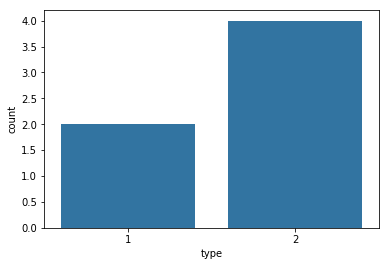``````# order by type count
type_order = df_ai_t['type'].value_counts().index
sb.countplot(data = df_ai_t, x = 'type', order=type_order, color=sb.color_palette());
``````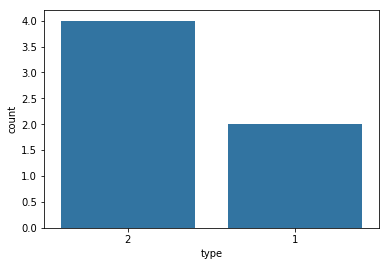## Histograms

### matplotlib.pyplot.hist

Using histograms for univariate quantitative variables using matplotlib.pyplot.hist.

``````# the dataset
df_ai_t
``````
bending dangerous humor id intellect type
ava 10 95 1 9272 80 2
bender 100 50 90 7912 20 2
hal 20 90 1 70 100 1
johnny 5 2 12 5 836 1 2
rachael 1 40 1 7192 80 2
wintermute 1 100 1 4030 100 1

Plotting the dangerous attribute of our bots into a histogram.

``````plt.hist(data = df_ai_t, x = 'dangerous', bins = 4);
plt.xlabel('Danger Range')
plt.ylabel('Number of Bots');
``````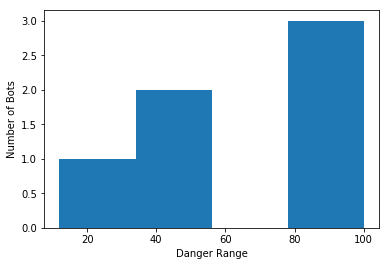### seaborn.distplot

Using seaborn.distplot to visualize a univariate distribution of bot humor observations.

``````sb.distplot(df_ai_t['humor']);
``````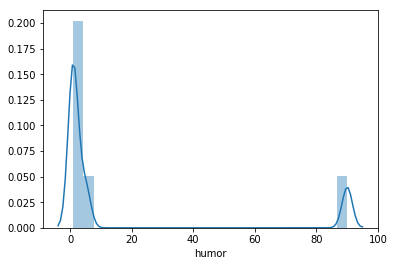### matplotlib.pyplot.hist2d

A 2D histogram using matplotlib.pyplot.hist2d correlating bending with humor.

``````plt.hist2d(data=df_ai_t, x='bending', y='humor')
plt.colorbar()
plt.xlabel('Bending Ability')
plt.ylabel('Humor');
``````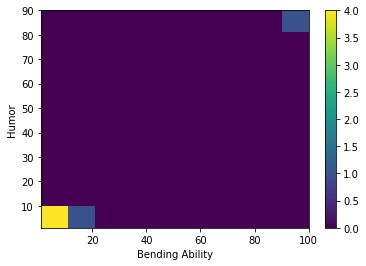## Scatterplots

Using scatterplots for bivariate visulations of quantitative vs quantitative data.

``````# the dataset
df_ai_t
``````
bending dangerous humor id intellect type
ava 10 95 1 9272 80 2
bender 100 50 90 7912 20 2
hal 20 90 1 70 100 1
johnny 5 2 12 5 836 1 2
rachael 1 40 1 7192 80 2
wintermute 1 100 1 4030 100 1

### matplotlib.pyplot.scatter

Statistically quantifying the strength of linear correlation between humor and bending using matplotlib.pyplot.scatter.

``````plt.scatter(data = df_ai_t, marker="o", color="red", x='bending', y='humor');
plt.xlabel('Bending Ability')
plt.ylabel('Humor');
``````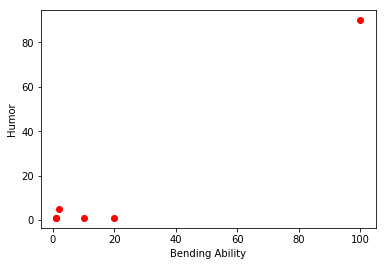### seaborn.regplot

A linear regression model fit of humor and bending using seaborn.regplot.

``````sb.regplot(data = df_ai_t, marker="o", color="red", x='bending', y='humor');
plt.xlabel('Bending Ability')
plt.ylabel('Humor');
``````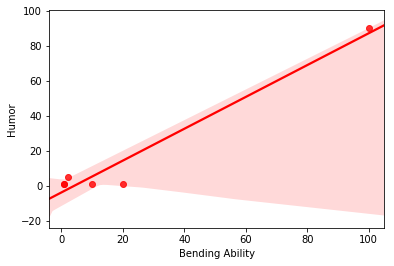## Subplots

### matplotlib.pyplot.subplots

Visualizing correlations of bending, intellect and dangerous to the shared y-axis humor using matplotlib.pyplot.subplots.

``````fig, (ax1, ax2, ax3) = plt.subplots(nrows=1, ncols=3, sharey=True)

sb.regplot(ax=ax1, data = df_ai_t, marker="o", color="red", x='bending', y='humor');
sb.regplot(ax=ax2, data = df_ai_t, marker="o", color="red", x='intellect', y='humor');
sb.regplot(ax=ax3, data = df_ai_t, marker="o", color="red", x='dangerous', y='humor');
``````## Resources

Python Data Essentials - Matplotlib and Seaborn: A beginners guide. by Craig Johnston is licensed under a Creative Commons Attribution 4.0 International License.